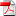# A Probabilistic Numerical Method for Fully Nonlinear Parabolic PDEs

1
Nov

### Arash Fahim, Nizar Touzi, Xavier Warin

We consider the probabilistic numerical scheme for fully nonlinear PDEs suggested in , and show that it can be introduced naturally as a combination of Monte Carlo and finite differences scheme without appealing to the theory of backward stochastic differential equations. Our first main result provides the convergence of the discrete-time approximation and derives a bound on the discretization error in terms of the time step. An explicit implementable scheme requires to approximate the conditional expectation operators involved in the discretization. This induces a further Monte Carlo error. Our second main result is to prove the convergence of the latter approximation scheme, and to derive an upper bound on the approximation error. Numerical experiments are performed for the approximation of the solution of the mean curvature ow equation indimensions two and three, and for two and five-dimensional (plus time) fully-nonlinear Hamilton-Jacobi-Bellman equations arising in the theory of portfolio optimization in financial mathematics.

Plus d'infos.

•rr-fime-09-05